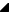﻿ TRSPieChart.Offset Property# TRSPieChart.Offset Property

Defines where on the pie chart the first pie slice starts. By default (Offset = 0), the pie chart draws the first pie slice starting to the right (3:00 on a clock) and goes counter-clockwise. By specifying a different offset from 0 to 359, the first pie slice will start somewhere else on the clock (90=12:00, 180=9:00, etc).

Namespace: FMX.RS.ChartsSyntax
 Delphi published   property Offset: Integer read FOffset write SetOffset default 0;

Property Value

Type: IntegerExamples
 The following code adds 3 pie slices to the chart: Delphi var    Value: TRSPieChartValue;  // note, this type should be the same that the chart uses (e.g., TRS2DChartValue, TRSArrowChartValue, etc)  begin    // Add first point    Value := RSPieChart1.Values.Add;    Value.Color := clRed;    Value.Value := 10.0;    // Add second point, twice as large as first    Value := RSPieChart1.Values.Add;    Value.Color := clGreen;    Value.Value := 20.0;    // Add third point, twice as large as second    Value := RSPieChart1.Values.Add;    Value.Color := clNavy;    Value.Value := 40.0;      // Draw the pie slices starting at 12:00 and go counter-clockwise    RSPieChart1.Offset := 90;  end;See Also

#### Reference

 • FMX.RS.Charts.TRSPieChart.Values
 • FMX.RS.Charts.TRSPieChartValues
 • TRSPieChartValue.Value
 • TRSPieChart
 • FMX.RS.Charts

RiverSoftAVG Charting Component Suite (RCCS) © 2005-2015, Thomas G. Grubb